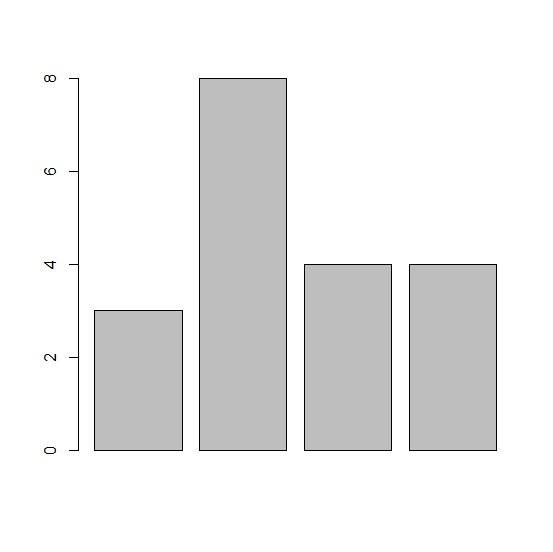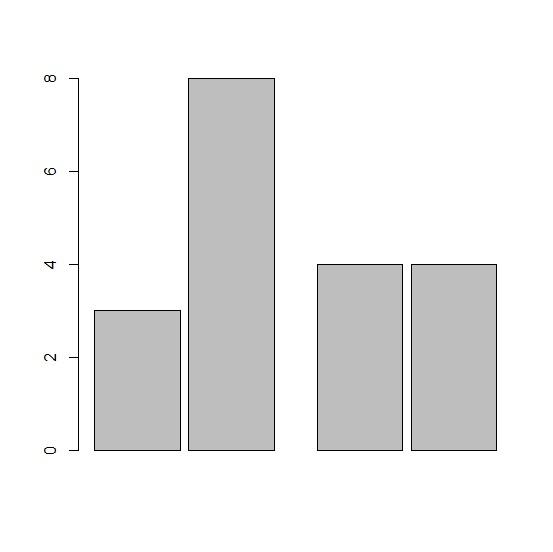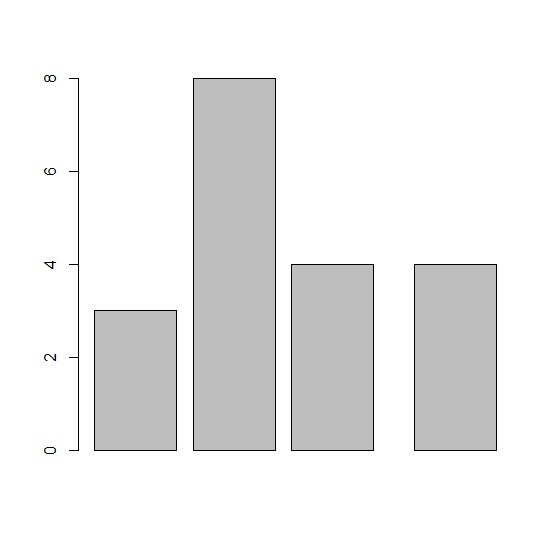# How to change the space between bars in a barplot in R?

R ProgrammingServer Side ProgrammingProgramming

#### Artificial Intelligence : The Future Of Programming

15 Lectures 54 mins

#### Beyond Basic Programming - Intermediate Python

Most Popular

36 Lectures 3 hours

#### C Programming from scratch- Master C Programming

Best Seller

60 Lectures 8 hours

By default, the space between bars is equal irrespective of the number of bars in the plot. If we want to have different space between bars then space arguments need to be used inside the barplot function but the first value does not make an impact because the first space is fixed between Y-axis and the first bar. For example, if we have a vector x that contains three values then the barplot with different space between bars can be created by using the below command −

barplot(x,space=c(0.5,0.1,0.5))

## Example

Live Demo

x<-rpois(4,5)
x

## Output

 3 8 4 4

## Example

barplot(x)

## Output## Example

barplot(x,space=c(0.01,0.1,0.5,0.1))


## Output## Example

barplot(x,space=c(0.1,0.2,0.2,0.5))

## Output